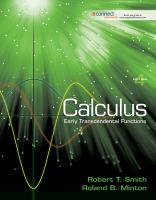Close

# Loose Leaf Version for Calculus Early Transcendental Functions

## by Smith, Robert, Minton, Roland• ISBN: 9780077431396
• ISBN10: 0077431391

# Loose Leaf Version for Calculus Early Transcendental Functions

## by Smith, Robert, Minton, Roland

• List Price: \$195.67
• Binding: Ring-bound
• Edition: 4
• Publisher: McGraw-Hill
• Publish date: 01/05/2011
• ISBN: 9780077431396
• ISBN10: 0077431391
new \$188.14
You save: 4%
FREE economy shipping!
Description: Calculus: Early Transcendental Functions Chapter 0: Preliminaries 0.1, "Polynomials and Rational Functions" 0.2, "Graphing Calculators and Computer Algebra Systems" 0.3, "Inverse Functions" 0.4, "Trigonometric and Inverse Trigonometric Functions" 0.5, "Exponential and Logarithmic Functions" 0.6, "Transformations of Functions" Chapter 1: Limits and Continuity 1.1, "A Brief Preview of Calculus: Tangent Lines and the Length of a Curve 1.2, "The Concept of Limit" 1.3, "Computation of Limits" 1.4, "Continuity and Its Consequences" 1.5, "Limits Involving Infinity; Asymptotes" 1.6, "Formal Definition of the Limit" 1.7, "Limits and Loss-of-Significance Errors" Chapter 2: Differentiation 2.1, "Tangent Lines and Velocity" 2.2, "The Derivative" 2.3, "Computation of Derivatives: The Power Rule" 2.4, "The Product and Quotient Rules" 2.5, "The Chain Rule" 2.6, "Derivatives of Trigonometric Functions" 2.7, "Derivatives of Exponential and Logarithmic Functions" 2.8, "Implicit Differentiation and Inverse Trigonometric Functions" 2.9, "The Hyperbolic Functions" 2.10, "The Mean Value Theorem" Chapter 3: Applications of the Derivative 3.1, "Linear Approximations and Newton''s Method"" 3.2, "Indeterminate Forms and L''Hpital''s Rule " 3.3, "Maximum and Minimum Values" 3.4, "Increasing and Decreasing Functions" 3.5, "Concavity and the Second Derivative Test" 3.6, "Overview of Curve Sketching" 3.7, "Optimization" 3.8, "Related Rates" 3.9, "Rates of Change in Economics and the Sciences" Chapter 4: Integration 4.1, "Antiderivatives" 4.2, "Sums and Sigma Notation" 4.3, "Area" 4.4, "The Definite Integral" 4.5, "The Fundamental Theorem of Calculus" 4.6, "Integration by Substitution" 4.7, "Numerical Integration" 4.8, "The Natural Logarithm as an Integral" Chapter 5: Applications of the Definite Integral 5.1, "Area Between Curves" 5.2, "Volume: Slicing, Disks and Washers" 5.3, "Volumes by Cylindrical Shells" 5.4, "Arc Length and Surface Area" 5.5, "Projectile Motion" 5.6, "Applications of Integration to Physics and Engineering" 5.7, "Probability" Chapter 6: Integration Techniques 6.1, "Review of Formulas and Techniques" 6.2, "Integration by Parts" 6.3, "Trigonometric Techniques of Integration" 6.4, "Integration of Rational Functions Using Partial Fractions" 6.5, "Integration Tables and Computer Algebra Systems" 6.6, "Improper Integrals" Chapter 7: First-Order Differential Equations 7.1, "Modeling with Differential Equations" 7.2, "Separable Differential Equations" 7.3, "Direction Fields and Euler''s Method" 7.4, "Systems of First-Order Differential Equations" Chapter 8: Infinite Series 8.1, "Sequences of Real Numbers" 8.2, "Infinite Series" 8.3, "The Integral and Comparison Tests" 8.4, "Alternating Series" 8.5, "Absolute Convergence and the Ratio Test" 8.6, "Power Series" 8.7, "Taylor Series" 8.8, "Applications of Taylor Series" 8.9, "Fourier Series" Chapter 9: Parametric Equations and Polar Coordinates 9.1, "Plane Curves and Parametric Equations" 9.2, "Calculus and Parametric Equations" 9.3, "Arc Length and Surface Area in Parametric Equations" 9.4, "Polar Coordinates" 9.5, "Calculus and Polar Coordinates" 9.6, "Conic Sections" 9.7, "Conic Sections in Polar Coordinates" Chapter 10: Vectors and the Geometry of Space 10.1, "Vectors in the Plane" 10.2, "Vectors in Space" 10.3, "The Dot Product" 10.4, "The Cross Product" 10.5, "Lines and Planes in Space" 10.6, "Surfaces in Space" Chapter 11: Vector-Valued Functions 11.1, "Vector-Valued Functions" 11.2, "The Calculus of Vector-Valued Functions" 11.3, "Motion in Space" 11.4, "Curvature" 11.5, "Tangent and Normal Vectors" 11.6, "Parametric Surfaces" Chapter 12: Functions of Several Variables and Partial Differentiation 12.1, "Functions of Several Variables" 12.2, "Limits and Continuity" 12.3, "Partial Derivatives" 12.4, "Tangent Planes and Linear Approximations" 12.5, "The Chain Rule" 12.6, "The Gradient and Directional Derivatives" 12.7, "Extrema of Functions of Several Variables" 12.8, "Constrained Optimization and and Lagrange Multipliers" Chapter 13: Multiple Integrals 13.1, "Double Integrals" 13.2, "Area, Volume and Center of Mass" 13.3, "Double Integrals in Polar Coordinates" 13.4, "Surface Area" 13.5, "Triple Integrals" 13.6, "Cylindrical Coordinates" 13.7, "Spherical Coordinates" 13.8, "Change of Variables in Multiple Integrals" Chapter 14: Vector Calculus 14.1, "Vector Fields" 14.2, "Line Integrals" 14.3, "Independence of Path and Conservative Vector Fields" 14.4, "Green''s Theorem" 14.5, "Curl and Divergence" 14.6, "Surface Integrals" 14.7, "The Divergence Theorem" 14.8, "Stokes'' Theorem" 14.9, "Applications of Vector Calculus" Chapter 15: Second Order Differential Equations 15.1, Second-Order Equations With Constant Coefficients" 15.2, "Nonhomogeneous Equations: Undetermined Coefficients" 15.3, "Applications of Second-Order Equations" 15.4, "Power Series Solutions of Differential Equations" Appendix A: Proofs of Selected Theorems Appendix B: Answers to Odd-Numbered Exercises
Expand description